Mathematics
Multiplication and Division Tricks
29.1k views

 1 Introduction 2 VWhat are the different Vedic Maths Sutras? 3 Multiplication Tricks! Get quicker at calculations 4 Division Tricks! Get quicker at calculations 5 Summary 6 FAQs

13th November 2020

Reading Time: 15 Minutes

## Introduction

Do you want to Multiply and Divide 10 times faster?

Are you struggling with mental calculations?

Vedic Mathematics is a collection of Formulae/Techniques called Sutras which help us solve arithmetic problems. The techniques are said to have been taken from multiple Sanskrit texts. The Sutras in Vedic Mathematics were put together by Indian Mathematician Shri Bharati Krishna Tirthaji around the early 20th century.
We will see more about the techniques suggested in Vedic Mathematics in the following sections.

## What are the different Vedic Maths sutras?

Vedic Mathematics gained its popularity because of its different techniques which help in speedy and accurate calculations.

The Sutras help us solve arithmetic problems in simpler and faster ways.

There are 16 Sutras and 13 Sub-Sutras.

Names of the 16 main Sutras are listed here.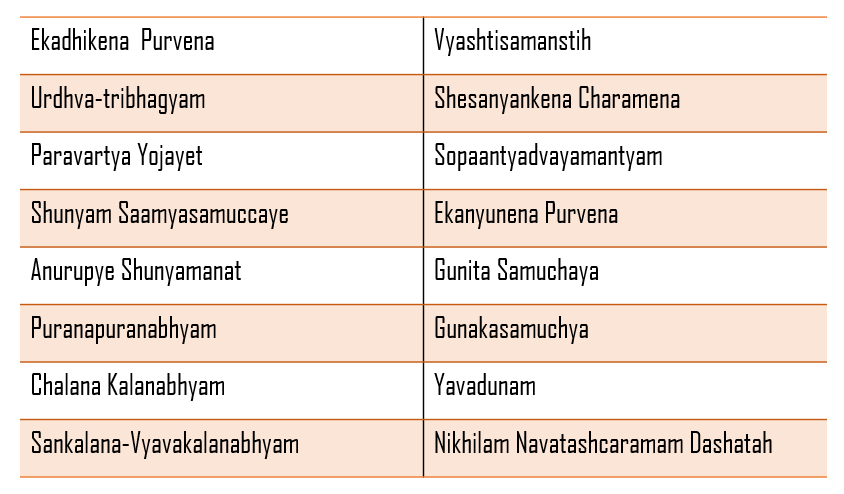We will see more about specific techniques in the next section.

## Multiplication Tricks! Get quicker at calculations

In this section, we will see a few multiplication tricks listed in the Sutras.

### Multiplication of a 2-digit number from 11 to 20

Step 1: Add the larger number to the unit digit of the smaller number

Step 2: Multiply the answer obtained from step 1 by 10

Step 3: Multiply the unit digits of both the numbers

Step 4: Add the answers from Step 2 and Step 3

Let us see an example.

Multiply 14 × 18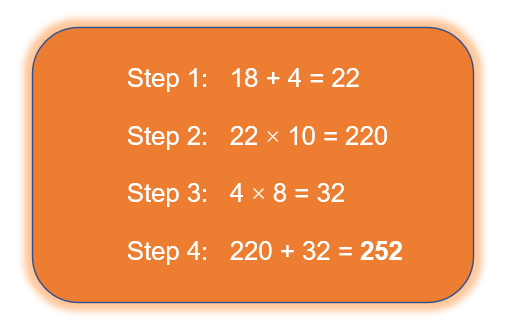### (Nikhilam)

This can be further divided into two categories.

• Numbers lesser/greater and closer to the power of 10 (both numbers on the same side to the power of 10)
• Numbers closer and lying on both sides to the power of 10

### Numbers lesser/greater and closer to the power of 10 (on the same side)

Step 1: Find the difference between the numbers from the closest power of 10 and multiply them.

Step 2: Add one of the two given numbers to the difference of the other number from the closest power of 10 (Subtract the closest power of 10 from the number)

Step 3: Final answer is obtained by joining the answer from step 2 and step 1

Example

Multiply 93 × 96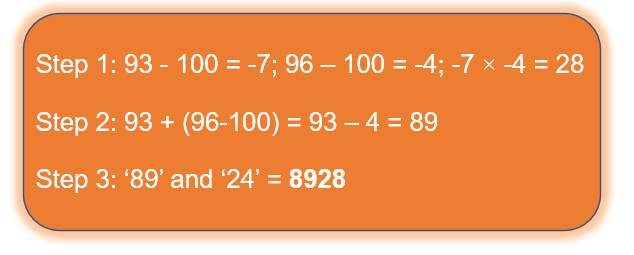### Numbers closer and lying on both sides to the power of 10

Step 1: Find the difference between the numbers from the closest power of 10 and multiply them.

Step 2: Add one of the two given numbers to the difference of the other number from the closest power of 10

Step 3: Final answer is obtained by joining the answer from step 2 and step 1 using the Vinculum method

### Vinculum numbers are the basis for Vedic Mathematics.

What is Vinculum?

Vinculum Method

We know that any number can be written in its expanded form based on the digits’ place values.

For example,

2456 can be written as 2000 + 400 + 50 + 6. This is one method of expansion.

Can we write 2456 in a different manner?

Yes. For instance, 2456 can also be written as 2500 – 44

In this case, it is represented as \begin{align}25\overline {44} \end{align} in the Vinculum method. They are called Bar numbers. A bar is seen on top of the negative part of the numbers.

This number \begin{align}25\overline {44} \end{align}  is expanded as: 2000 + 500 - 40 – 4 = 2456

Let us try to solve the multiplication of 2 numbers lying on either side to the power of 10.

Multiply 97 × 104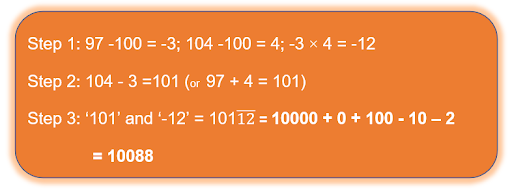### (Ekayunena Purvena)

The method is employed when one of the numbers consists of all 9s.
There are subcategories in this method.

When the number of digits in both the multiplier (number with 9s) and the multiplicand is the same

Step 1: The first part of the answer is one less than the multiplicand

Step 2: The second part of the answer is the complement of the multiplicand

Let us see an example.

Multiply 22 × 99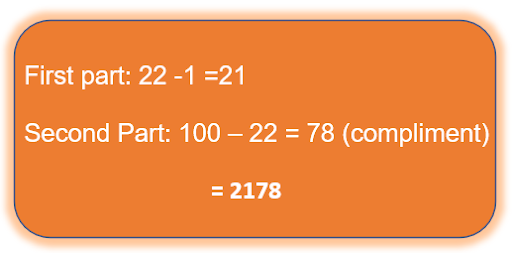When the number of digits in the multiplier (number with 9s) is less than the multiplicand

Step 1: If the multiplicand starts with 1, subtract 2 from the whole number. If the multiplicand starts with 2, subtract 3 from it and so on. This is the first part of the answer.

Step 2: This is the compliment of the last digit of the multiplicand.

Let us see an example.

Multiply 34 × 9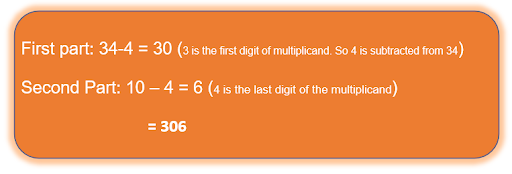When the number of digits in the multiplier (number with 9s) is more than the multiplicand

Step 1: The first part of the answer is 1 less than the multiplicand

Step 2: The second part of the answer is 9

Step 3: Compliment of the multiplicand

Example

Multiply 4 × 99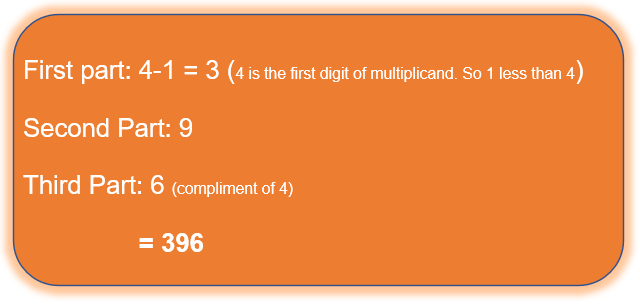### Square of numbers ending with 5

Step 1: First part of the answer is the product of the digits in the number (excluding 5) and its next number.

Step 2: The second part of the number is always 25

Example

Square of 75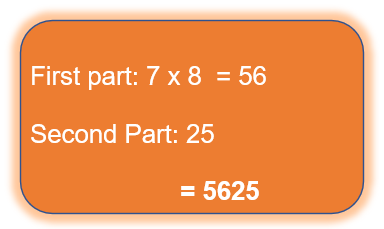### (Anurupyena)

When two numbers that are close to each other are multiplied, the following technique can be employed.

Step 1: Consider the base as the nearest power of 10. For example, if the number is close to 80, we should take the base as 80 (8 ×10). Here the factor is 8. If the number is close to 50, we should take the base as 50 (5 ×10 or 100 ÷ 2). Here the factor can be 5 or 2.

Step 2: Apply the same rules as those of Nikhilam

Step 3: Multiply or divide by the factor obtained in step 1

An example is shown here.

Multiply 84 × 85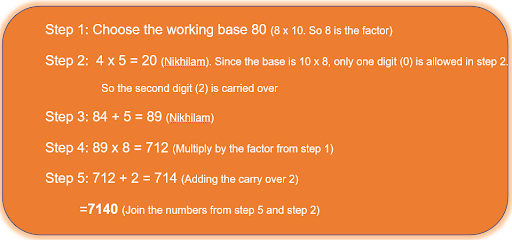## Division Tricks!  Get quicker at calculations

In this section we will learn few division tricks as explained in Vedic Math Sutras.

### (Nikhilam)

Step 1: Identify how much short the divisor is to the power of 10.

Step 2: Split the dividend into 2 parts – Quotient and Remainder. The number of digits considered as the remainder is the same as the number of digits in the divisor.

Step 3: Multiply the first digit of the quotient with the number obtained from step 1. Add this to the unit’s digit of the quotient part (from step 2).

Step 4: Multiply the number obtained from step 3 with the number from step 1. Add this number to the Remainder part (from step 2).

Step 5: Now, we check if the remainder is greater than or equal to the divisor. If yes, divide it again and add the new quotient part to the quotient (from step 2). The remainder obtained now is the final remainder.

Let us see an example below.

Divide 341 by 9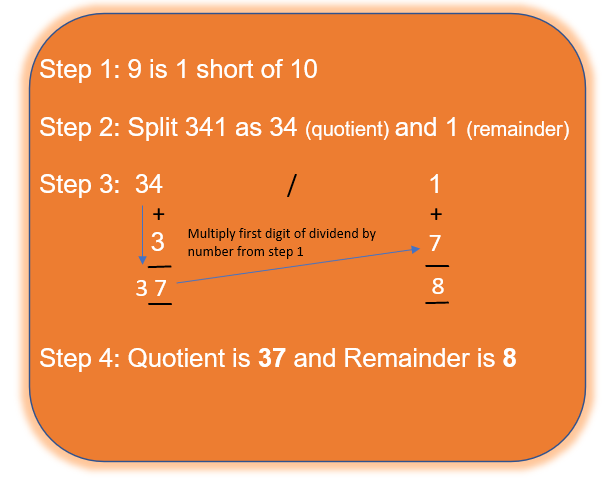### (Paravartya Sutra)

Step 1: Discard the first digit of the divisor and transpose the remaining digits of the divisor.

Step 2: Split dividend into 2 parts – Quotient and Remainder. The remainder should have the same number of digits as obtained in step 1.

Step 3: Follow the same steps as Nikhilam

If there is any Vinculum digit, solve the number accordingly (as explained previously in the article)

Let us see an example.

432 divided by 11### (Anurupyena Sutra)

Step 1: In order to convert the divisor so that Nikhilam method can be used, we multiply it by a factor which makes it closer to a power of 10

Step 2: Follow the same steps as Nikhilam

Step 3: Once the quotient and Remainder are obtained, multiply the quotient by the same factor used in step 1.

Step 4: Check if the remainder is bigger than or equal to the divisor and take appropriate action as explained in the Nikhilam method.

Let us see one example.

1011 divided by 23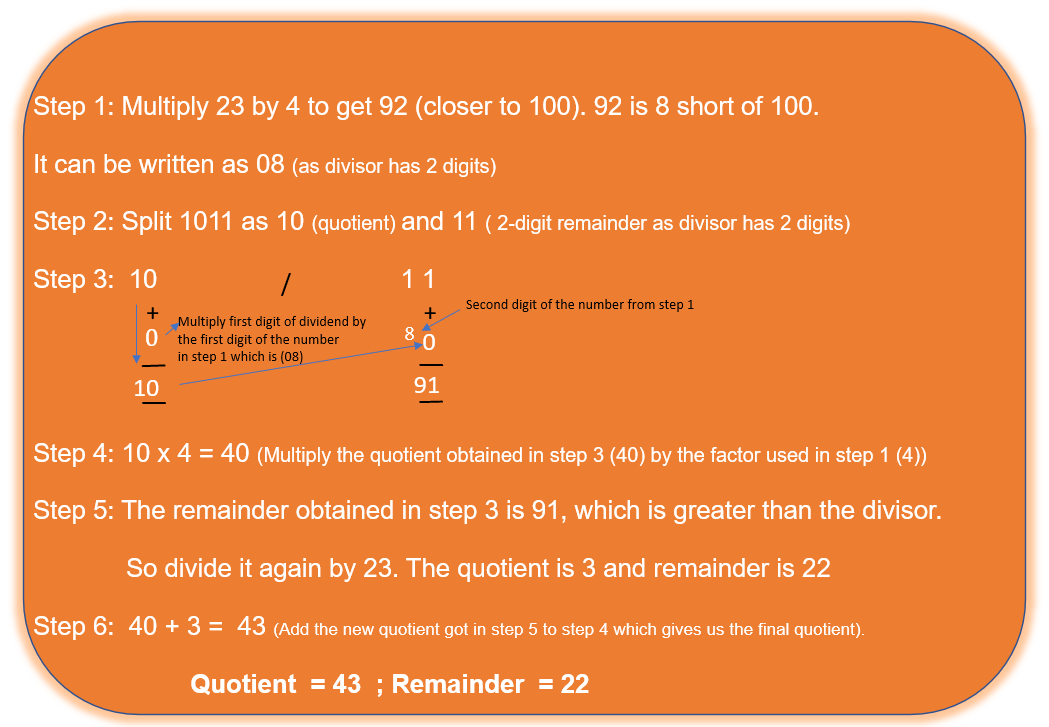## Summary

We have seen a few of the mathematical techniques available in Vedic Math in this article. There are many more techniques that are helpful in quickly solving polynomial equations, differential calculus, etc.

The main advantage of these techniques is that it helps the students spend less time on arithmetic calculations due to its clever tricks, which allows the students to focus more on the logical and reasoning parts of Mathematics.

This article was meant to be an initiator for Vedic Math. The students should explore more and make good use of these priceless tricks and benefit from them!

Cuemath, a student-friendly mathematics and coding platform, conducts regular Online Live Classes for academics and skill-development, and their Mental Math App, on both iOS and Android, is a one-stop solution for kids to develop multiple skills. Understand the Cuemath Fee structure and sign up for a free trial.

## Why is Vedic Math important?

Vedic Math has numerous techniques and rules that help us perform various arithmetic calculations in time. It reduces the calculating time from many minutes to very few seconds.

## Who created Vedic Maths?

The techniques in Vedic Math Indian Mathematician are said to have been put together by Shri Bharati Krishna Tirthaji. It is said to have been obtained from Atharva Veda.

## What does Vedic mean?

‘Veda’ is a Sanskrit word and means ‘Knowledge’.

## When was Vedic Maths discovered?

Shri Bharati Krishna Tirthaji compiled all these techniques in the early 20th century.

## How do you multiply faster in Vedic Maths?

The techniques suggested in Vedic Math were carefully formed considering various scenarios and combination of numbers that are possible. A variety of categories and the appropriate steps have been listed down neatly in the rule book. Please refer to the Multiplication Tricks section for more information on this.